Win up to 100% scholarship on Aakash BYJU'S JEE/NEET courses with ABNAT Win up to 100% scholarship on Aakash BYJU'S JEE/NEET courses with ABNAT

# JEE Main 2022 June 25 – Shift 1 Chemistry Question Paper with Solutions

Students can access the JEE Main 2022 June 25 Shift 1 Chemistry Question Paper and Solutions here. Our subject experts have provided answers for all the JEE main 2022 question papers in an easily understandable way, to help students refer to them at any time, and download the PDF, if required. Practising and solving multiple JEE main chemistry question papers will help students understand the difficulty level and pattern in the exam. Get solutions for Session 1 – June 25 Shift 1 – chemistry question paper of JEE main 2022 here on this page.
JEE Main 2022 June 25 – Shift 1 Chemistry Question Paper with Solutions

SECTION – A

Multiple Choice Questions: This section contains 20 multiple choice questions. Each question has 4 choices (1), (2), (3) and (4), out of which ONLY ONE is correct.

1. Bonding in which of the following diatomic molecule(s) become(s) stronger, on the basis of MO Theory, by removal of an electron?

(A) NO

(B) N2

(C) O2

(D) C2

(E) B2

Choose the most appropriate answer from the options given below :

(A) (A), (B), (C) only

(B) (B), (C), (E) only

(C) (A), (C) only

(D) (D) only

Sol. If an electron is removed from the anti-bonding orbital, then it will tend to increase the bond order.

The HOMO in NO and O2 is antibonding molecular orbital .

Hence, in NO and O2 bond order will increase on loss of electron.

2. Incorrect statement for Tyndall effect is :

(A) The refractive indices of the dispersed phase and the dispersion medium differ greatly in magnitude.

(B) The diameter of the dispersed particles is much smaller than the wavelength of the light used.

(C) During projection of movies in the cinemas hall, Tyndall effect is noticed.

(D) It is used to distinguish a true solution from a colloidal solution.

Sol. For Tyndall effect, the diameter of the dispersed particles is not much smaller than the wavelength of the light used.

3. The pair, in which ions are isoelectronic with Al3+ is:

(A) Br and Be2+

(B) Cl and Li+

(C) S2– and K+

(D) O2– and Mg2+

Sol. O2–, Mg2+ and Al3+ are isoelectronic. All have 10 electrons.

4. Leaching of gold with dilute aqueous solution of NaCN in presence of oxygen gives complex [A], which on reaction with zinc forms the elemental gold and another complex [B]. [A] and [B], respectively are :

(A) [Au(CN)4] and [Zn(CN)2 (OH)2]2-

(B) [Au(CN)2] and [Zn (OH)4]2-

(C) [Au(CN)2] and [Zn (CN)4]2-

(D) [Au(CN)4]2- and [Zn (CN)6]4-

Sol. In the metallurgy of gold

4Au + 8CN + 2H2O + O2 → 4[Au(CN)2] + 4OH

2[Au(CN)2] + Zn → [Zn(CN)4]2– + 2Au

5. Number of electron deficient molecules among the following

PH3, B2H6, CCl4, NH3, LiH and BCl3 is

(A) 0

(B) 1

(C) 2

(D) 3

Sol. Only B2H6 and BCl3 are e deficient among the given molecules.

6. Which one of the following alkaline earth metal ions has the highest ionic mobility in its aqueous solution?

(A) Be2+

(B) Mg2+

(C) Ca2+

(D) Sr2+

Sol. In aqueous solution, the ionic mobility is inversely proportional to the charge density on the ion. Hence Sr2+ has highest ionic mobility in water.

7. White precipitate of AgCI dissolves in aqueous ammonia solution due to formation of:

(A) [Ag(NH3)4]CI2

(B) [Ag(CI)2(NH3)2]

(C) [Ag(NH3)2]CI

(D) [Ag(NH3)CI]CI

Sol.

$$\begin{array}{l}AgCl+2NH_3\rightarrow\left [ Ag\left ( NH_3 \right )_2 \right ]Cl\end{array}$$

8. Cerium (IV) has a noble gas configuration. Which of the following is correct statement about it?

(A) It will not prefer to undergo redox reactions.

(B) It will prefer to gain electron and act as an oxidizing agent

(C) It will prefer to give away an electron and behave as reducing agent

(D) It acts as both, oxidizing and reducing agent.

Sol. E° value of Ce+4|Ce+3 is 1.74 V, which suggests that it is a very good oxidising agent.

9. Among the following which is the strongest oxidizing agent?

(A) Mn3+

(B) Fe3+

(C) Ti3+

(D) Cr3+

Sol.

$$\begin{array}{l}E^{\circ}_{Fe^{3+}|Fe^{2+}}=+0.77V\end{array}$$
$$\begin{array}{l}E^{\circ}_{Ti^{3+}|Ti^{2+}}=-0.37V\end{array}$$
$$\begin{array}{l}E^{\circ}_{Mn^{3+}|Mn^{2+}}=+1.57V\end{array}$$
$$\begin{array}{l}E^{\circ}_{Cr^{3+}|Cr^{2+}}=-0.41V\end{array}$$

Mn+3 is the best oxidising agent among the given series.

10. The eutrophication of water body results in:

(A) loss of Biodiversity.

(B) breakdown of organic matter.

(C) increase in biodiversity.

(D) decrease in BOD.

Sol. Eutrophication is the process in which nutrient enriched water bodies support a dense plant population, which kills animal life by depriving it of oxygen and results in subsequent loss of biodiversity.

11. Phenol on reaction with dilute nitric acid, gives two products. Which method will be most efficient for large scale separation?

(A) Chromatographic separation

(B) Fractional crystallisation

(C) Steam distillation

(D) Sublimation

Sol.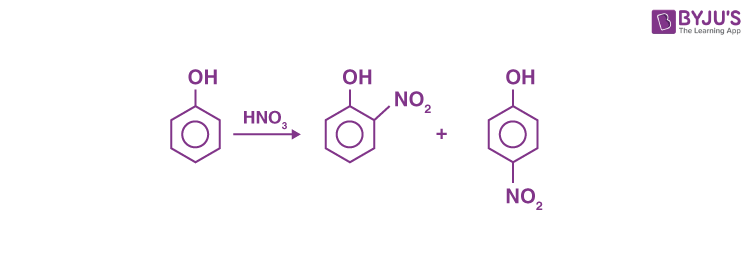o-Nitrophenol and p-Nitrophenol can be easily separated by steam distillation.

12. In the following structures, which one is having staggered conformation with maximum dihedral angle?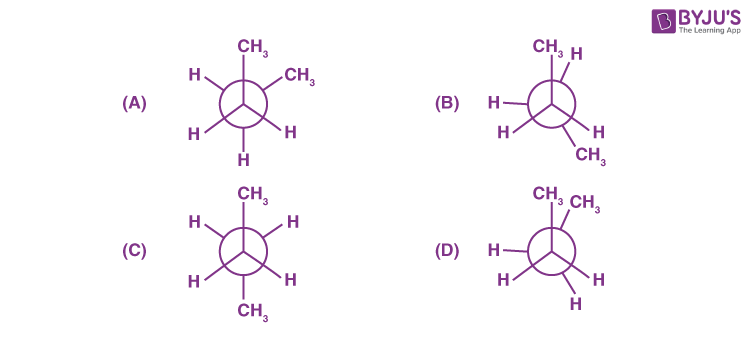Sol.It is the staggered conformation with maximum dihedral angle.

13. The product formed in the following reaction.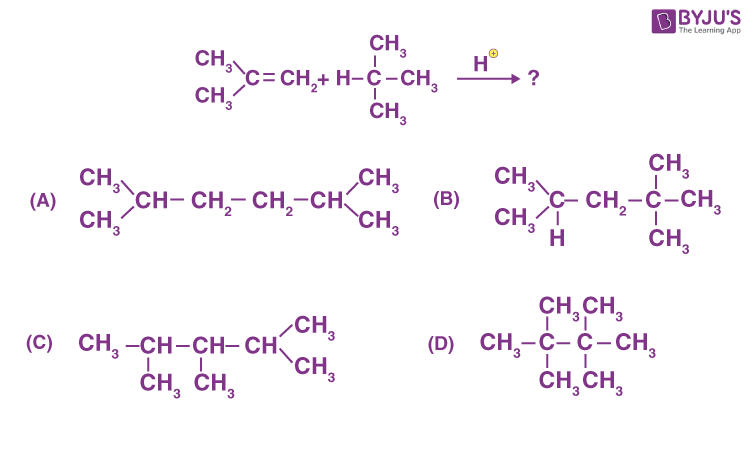Sol.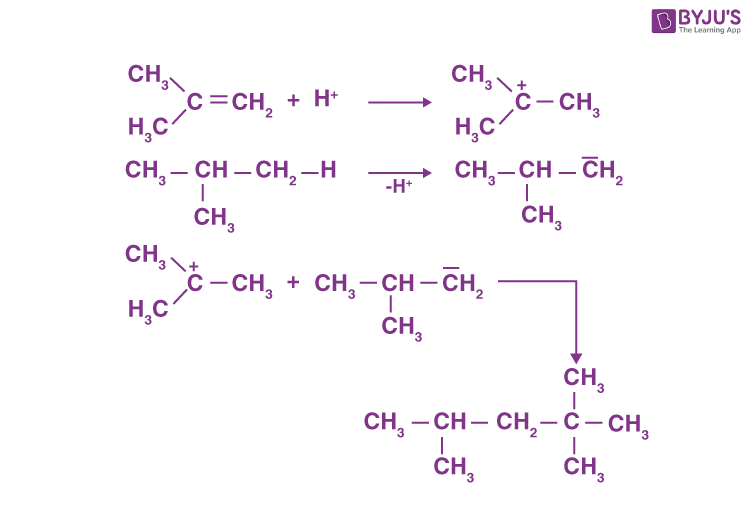14. The IUPAC name of ethylidene chloride is:

(A) 1-Chloroethene

(B) 1-Chloroethyne

(C) 1, 2-Dichloroethane

(D) 1, 1-Dichloroethane

Sol. Ethylidene chloride is CH3 – CHCl2, its IUPAC name is 1,1-Dichloromethane.

15. The major product in the reactionis:

(A) t-Butyl ethyl ether

(B) 2, 2-Dimethyl butane

(C) 2-Methyl pent-1-ene

(D) 2-Methyl prop-1-ene

Sol.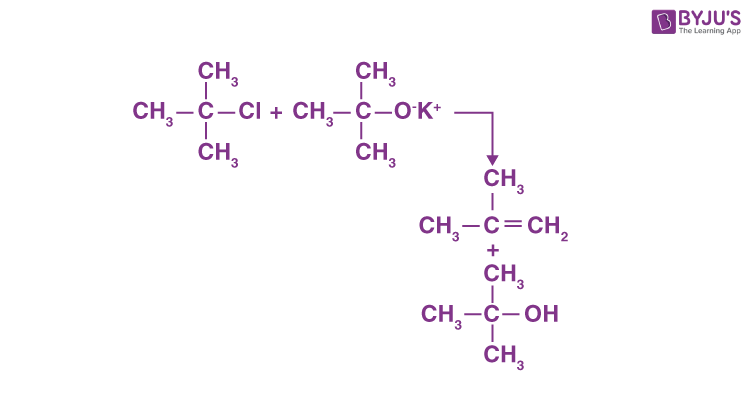16. The intermediate X, in the reaction :is :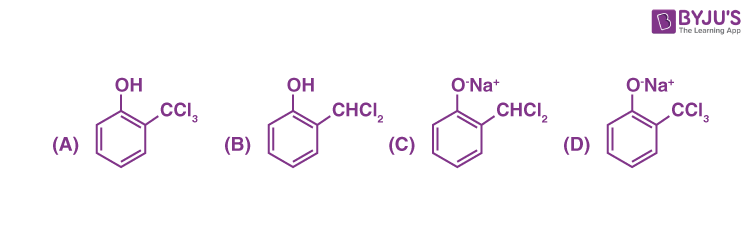Sol.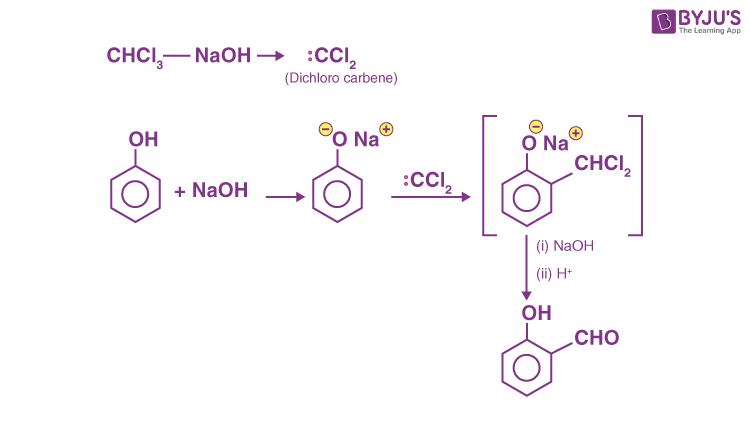17. In the following reaction: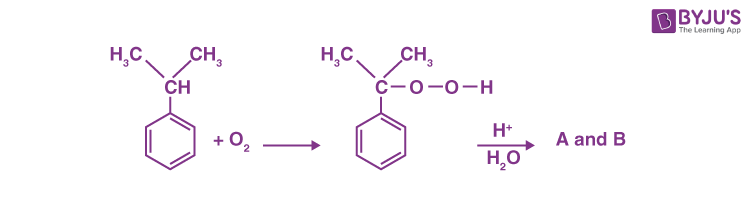The compound A and B respectively are: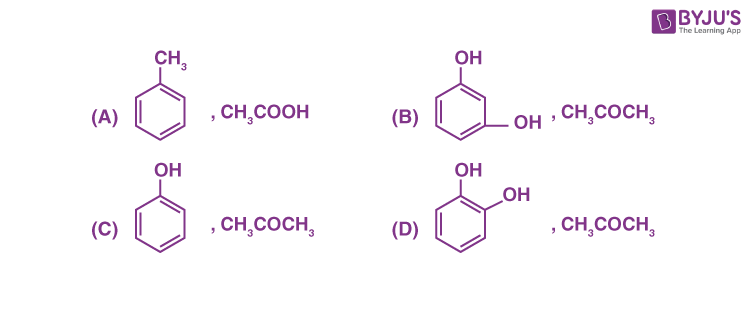Sol.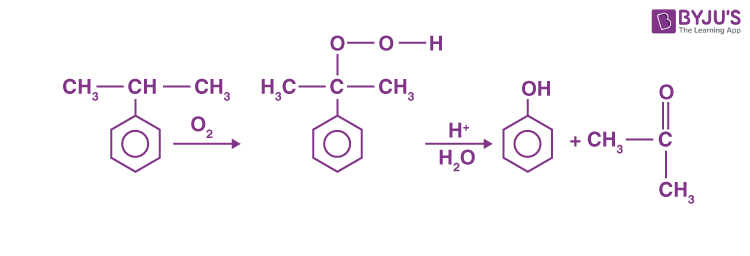18. The reaction of

$$\begin{array}{l}R-\underset {\underset{{O}}{||}}{C}-NH_2\end{array}$$
with bromine and KOH gives RNH2 as the end product. Which one of the following is the intermediate product formed in this reaction?

(A)

$$\begin{array}{l}R-\underset {\underset{{O}}{||}}{C}-NH-Br\end{array}$$

(B) R – NH – Br

(C) R – N = C = O

(D)

$$\begin{array}{l}R-\underset {\underset{{O}}{||}}{C}-NBr_2\end{array}$$

Sol.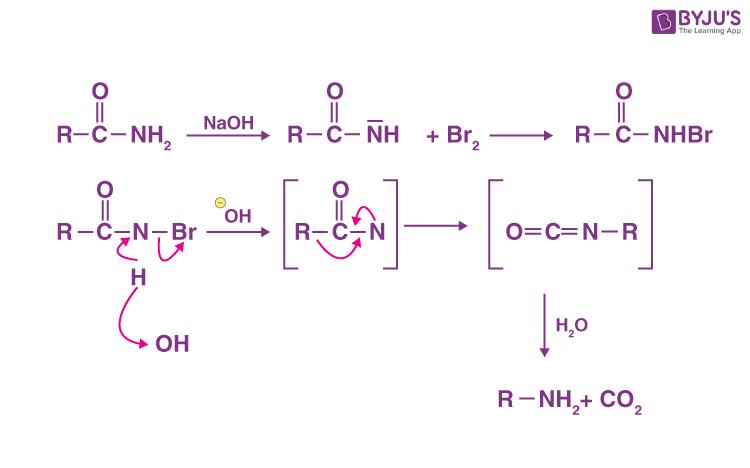19. Using very little soap while washing clothes, does not serve the purpose of cleaning of clothes, because:

(A) soap particles remain floating in water as ions.

(B) the hydrophobic part of soap is not able to take away grease.

(C) the micelles are not formed due to concentration of soap, below its CMC value.

(D) colloidal structure of soap in water is completely distributed.

Sol. The little amount of using soap while washing clothes, does not serve the purpose of cleaning of clothes because the micelles are not formed due to the low concentration of soap as it is below CMC.

20. Which one of the following is an example of artificial sweetner?

(A) Bithional

(B) Alitame

(C) Salvarsan

(D) Lactose

Sol. Alitame is an artificial sweetner.

SECTION – B

Numerical Value Type Questions: This section contains 10 questions. In Section B, attempt any five questions out of 10. The answer to each question is a NUMERICAL VALUE. For each question, enter the correct numerical value (in decimal notation, truncated/rounded-off to the second decimal place; e.g. 06.25, 07.00, –00.33, –00.30, 30.27, –27.30) using the mouse and the on-screen virtual numeric keypad in the place designated to enter the answer.

1. The number of N atoms in 681 g of C7H5N3O6 is x × 1021. The value of x is ______. (NA = 6.02 × 1023 mol–1) (Nearest Integer)

Sol. Molar mass of C7H5N3O6 = 227 g/mol

681 g of C7H5N3O6 = 3 mol

∴ 681 g of C7H5N3O6 has 9 mole of N.

= 54.18 × 1023 N atoms

= 5418 × 1021

2. The distance between Na+ and Cl ions in solid NaCl of density 43.1 g cm–3 is ____ × 10–10 m. (Nearest Integer)

(Given : NA = 6.02 × 1023 mol–1)

Sol.

$$\begin{array}{l}\rho=\frac{Z\times M}{a^3\times N_A}\end{array}$$
$$\begin{array}{l}43.1=\frac{4\times58.5}{a^3\times6.02\times10^{23}}\end{array}$$

a3 = 0.9 × 10–23

= 9 × 10–24

a = 2.08 × 10–8 cm

= 2.08 × 10–10 m

for NaCl, distance between Na+ and Cl =

$$\begin{array}{l}\frac{a}{2}\end{array}$$

= 1.04 × 10–10 m

3. The longest wavelength of light that can be used for the ionisation of lithium atom (Li) in its ground state is x × 10–8 m. The value of x is ______. (Nearest Integer)

(Given : Energy of the electron in the first shell of the hydrogen atom is –2.2 x 10–18 J;
h = 6.63 × 10– 34 Js and c = 3 × 108 ms–1)

Sol. Energy required for ionisation of Li atom

= 2.2 × 10–18 ×

$$\begin{array}{l}\frac{9}{4}\end{array}$$
J [Assume this formula is True for Li atom]
$$\begin{array}{l}\because E=\frac{hc}{\lambda }\end{array}$$
$$\begin{array}{l}2.2\times10^{-18}\times\frac{9}{4}=\frac{6.63\times10^{-34}\times3\times10^8}{\lambda}\end{array}$$
$$\begin{array}{l}\lambda=4\times10^{-8}m\end{array}$$

4.The standard entropy change for the reaction 4Fe(s) + 3O2(g) 2Fe2O3(s) is –550 J K–1 at
298 K.

[Given: The standard enthalpy change for the reaction is –165 kJ mol–1]. The temperature in K at which the reaction attains equilibrium is ______. (Nearest Integer)

Sol.

$$\begin{array}{l}\because \Delta S=\frac{\Delta H}{T}\end{array}$$
$$\begin{array}{l}\therefore T=\frac{\Delta H}{\Delta S}\end{array}$$
$$\begin{array}{l}=\frac{165\times10^3}{550}\end{array}$$

= 300 K

5. 1 L aqueous solution of H2SO4 contains 0.02 m mol H2SO4. 50% of this solution is diluted with deionized water to give 1 L solution (A). In solution (A), 0.01 m mol of H2SO4 are added. Total m mols of H2SO4 in the final solution is ______ × 103 m mols.

Sol. Initially one litre contains 0.02 mole

∴ 50% of this solution will contains 0.01 m mol

After adding 0.01 mol, final solution will contain
0.02 m mol of H2SO4

= 0.02 m mol

Correct answer should be 0.02 × 10–3

6. The standard free energy change (ΔG°) for 50% dissociation of N2O4 into NO2 at 27°C and 1 atm pressure is –x J mol–1. The value of x is _____. (Nearest Integer)

[Given : R = 8.31 J K–1 mol–1, log 1.33 = 0.1239 ln 10 = 2.3]

Sol.

$$\begin{array}{l}N_2O_4\rightleftharpoons 2NO_2\end{array}$$

t = 0 1 0

t = teq. 1 – 0.5 2 × 0.5

$$\begin{array}{l}P_{N_2O_4}=0.33atm\end{array}$$
$$\begin{array}{l}P_{NO_2}=0.66~atm\end{array}$$
$$\begin{array}{l}K_p=\frac{\left ( P_{NO_2} \right )^2}{P_{N_2O_4}}=\frac{\left ( 0.66 \right )^2}{0.33}=1.33\end{array}$$

ΔG = –RTlnKp

= –8.31 × 300 × 2.3 × log1.33

$$\begin{array}{l}\approx 710\end{array}$$
Jmol–1

7. In a cell, the following reactions take place

$$\begin{array}{l}Fe^{2+}\rightarrow Fe^{3+}+e^-\end{array}$$
$$\begin{array}{l}E^\circ_{Fe^{3+}/Fe^{2+}}=0.77 V \end{array}$$
$$\begin{array}{l}2I^-\rightarrow I_2+2e^-\end{array}$$
$$\begin{array}{l}E_{I_2/I^-}=0.54V\end{array}$$

The standard electrode potential for the spontaneous reaction in the cell is x × 10–2 V at 208 K. The value of x is _______. (Nearest Integer)

Sol.

$$\begin{array}{l}E_{cell}^\circ=E_{cathode}^\circ-E_{anode}^\circ\end{array}$$

= 0.77 – 0.54

= 0.23 V

= 23 × 10–2 V

8. For a given chemical reaction

γ1A + γ2B → γ3C + γ4D

Concentration of C changes from 10 mmol dm–3 to 20 mmol dm–3 in 10 seconds. Rate of appearance of D is 1.5 times the rate of disappearance of B which is twice the rate of disappearance A. The rate of appearance of D has been experimentally determined to be 9 mmol dm–3 s–1. Therefore, the rate of reaction is _____ mmol dm–3 s–1.

(Nearest Integer)

Sol. Rate =

$$\begin{array}{l}\frac{1}{r_1}\left ( \frac{-d\left [ A \right ]}{dt} \right )=\frac{1}{r_2}\left ( \frac{-d\left [ B \right ]}{dt} \right )=\frac{1}{r_3}\left ( \frac{-d\left [ c \right ]}{dt} \right )=\frac{1}{r_4}\left ( \frac{d\left [ D \right ]}{dt} \right )\end{array}$$
$$\begin{array}{l}\frac{d\left [ D \right ]}{dt}=\frac{r_4}{r_2}\left ( \frac{-d\left [ B \right ]}{dt} \right )\end{array}$$
$$\begin{array}{l}\frac{r_4}{r_2}=\frac{3}{2}\end{array}$$
$$\begin{array}{l}\frac{-d\left [ B \right ]}{dt}=\frac{r_2}{r_1}\left ( \frac{-d\left [ A \right ]}{dt} \right )\Rightarrow \frac{r_2}{r_1}=2\end{array}$$

r4 = 1.5r2 = 3r1

$$\begin{array}{l}\frac{d\left [ C \right ]}{dt}\end{array}$$
= 1 m.mol dm–3 sec–1

$$\begin{array}{l}\frac{d\left [ D \right ]}{dt}\end{array}$$
= 9 m.mol dm–3 sec–1

$$\begin{array}{l}\frac{d\left [ D \right ]}{dt}=\frac{r_4}{r_3}\cdot\frac{d\left [ C \right ]}{dt}\Rightarrow\frac{r_4}{r_3}=9\end{array}$$

r4 = 9r3 = 3r1

⇒ r1 = 3r3

3r3A + 6r3B → r3C + 9r3D

∴ rate of reaction =

$$\begin{array}{l}\frac{1}{9}\times9\end{array}$$
m.mol dm–3 sec–1

= 1 m.mol dm–3 sec–1

9. If [Cu(H2O)4]2+ absorbs a light of wavelength 600 nm for d-d transition, then the value of octahedral crystal field splitting energy for [Cu(H2O)6]2+ will be _______ ×10–21 J. [Nearest integer]

(Given : h = 6.63 × 10–34 Js and c = 3.08 × 108 ms–1)

Sol. [Cu(H2O)4]2+ is tetrahedral

[Cu(H2O)6]2+ is octahedral

$$\begin{array}{l}\because \Delta_t=\frac{4}{9}\times\Delta_0\end{array}$$
$$\begin{array}{l}\Delta_t=\frac{6.63\times10^{-34}\times3.08\times10^8}{600\times10^{-9}} \end{array}$$
$$\begin{array}{l}\Delta_0=\frac{9}{4}\times\frac{6.63\times10^{-34}\times3.08\times10^8}{600\times10^{-9}}\end{array}$$
$$\begin{array}{l}\approx765\times10^{-21}J \end{array}$$

10. Number of grams of bromine that will completely react with 5.0 g of pent-1-ene is ______ × 10–2g. (Atomic mass of Br = 80 g/mol) [Nearest integer]

Sol.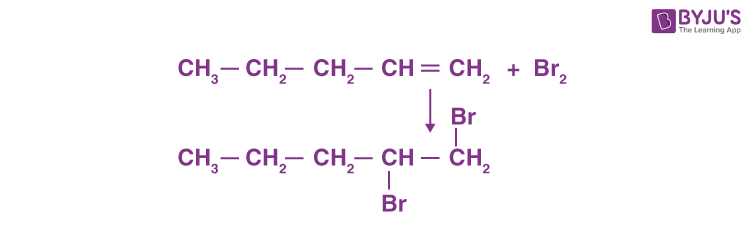$$\begin{array}{l}\frac{5}{70}\end{array}$$
moles of pentene will react with
$$\begin{array}{l}\frac{5}{70}\end{array}$$
moles of Br2

$$\begin{array}{l}=\frac{5}{70}\times160\end{array}$$

= 11.43 g

= 1143 × 10-2 g